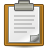Difference between revisions of "Template:Col-begin/doc"This is the template documentation page for {{Col-begin}}.It is not intended to be viewed directly. If it uses variables, some links may appear broken. Do not replace these variables with hardcoded page names or URLs.

This Template is a self-reference and so is part of Centiare rather than directory content.

Usage

A border of 2px (1px width on each side) corresponds to a 5%. Therefore, with a 2px border — like on Centiare:Tutorial (Wrap-up and more info) — the width needs to be 95% for the table to fit within the screen. Since it's possible that some tables might not have a border, correction on the individual page could be made after substitution. Another way do a two column table is like this:

 {{col-begin|width=95%}} |- | Left column | Right column |}
Template:Col-1-of-3 {{Col-begin}} {{Col-1-of-2}} Column 1 here {{Col-2-of-2}} Column 2 here {{Col-end}} Template:Col-2-of-3 {{Col-begin}} {{Col-1-of-3}} Column 1 here {{Col-2-of-3}} Column 2 here {{Col-3-of-3}} Column 3 here {{Col-end}} Template:Col-3-of-3 {{Col-begin}} {{Col-1-of-4}} Column 1 here {{Col-2-of-4}} Column 2 here {{Col-3-of-4}} Column 3 here {{Col-4-of-4}} Column 4 here {{Col-end}} Template:Col-end
Template:Col-1-of-3 {{Col-begin|width=}} {{Col-1-of-5}} Column 1 here {{Col-2-of-5}} Column 2 here {{Col-3-of-5}} Column 3 here {{Col-4-of-5}} Column 4 here {{Col-5-of-5}} Column 5 here {{Col-end}} Template:Col-2-of-3 {{Col-begin|bgColor=transparent}} {{Col-1-of-6}} Column 1 here {{Col-2-of-6}} Column 2 here {{Col-3-of-6}} Column 3 here {{Col-4-of-6}} Column 4 here {{Col-5-of-6}} Column 5 here {{Col-6-of-6}} Column 6 here {{Col-end}} Template:Col-3-of-3 {{Col-begin|class=references-small}} {{Col-break}} Column 1 here {{Col-break}} Column 2 here {{Col-break}} Column 3 here {{Col-break}} Column 4 here {{Col-break}} Column 5 here {{Col-break}} Column 6 here {{Col-end}} Template:Col-end
Template:Col-1-of-3 {{Top}} Column 1 here {{Mid}} Column 2 here {{Bottom}} Template:Col-2-of-3 {{Columns-start|num=3}} Column 1 here {{Column}} Column 2 here {{Column}} Column 3 here {{Columns-end}} Template:Col-3-of-3 {{Multicol}} Column 1 here {{Multicol-break}} Column 2 here {{Multicol-break}} Column 3 here {{Multicol-break}} Column 4 here {{Multicol-end}} Template:Col-end